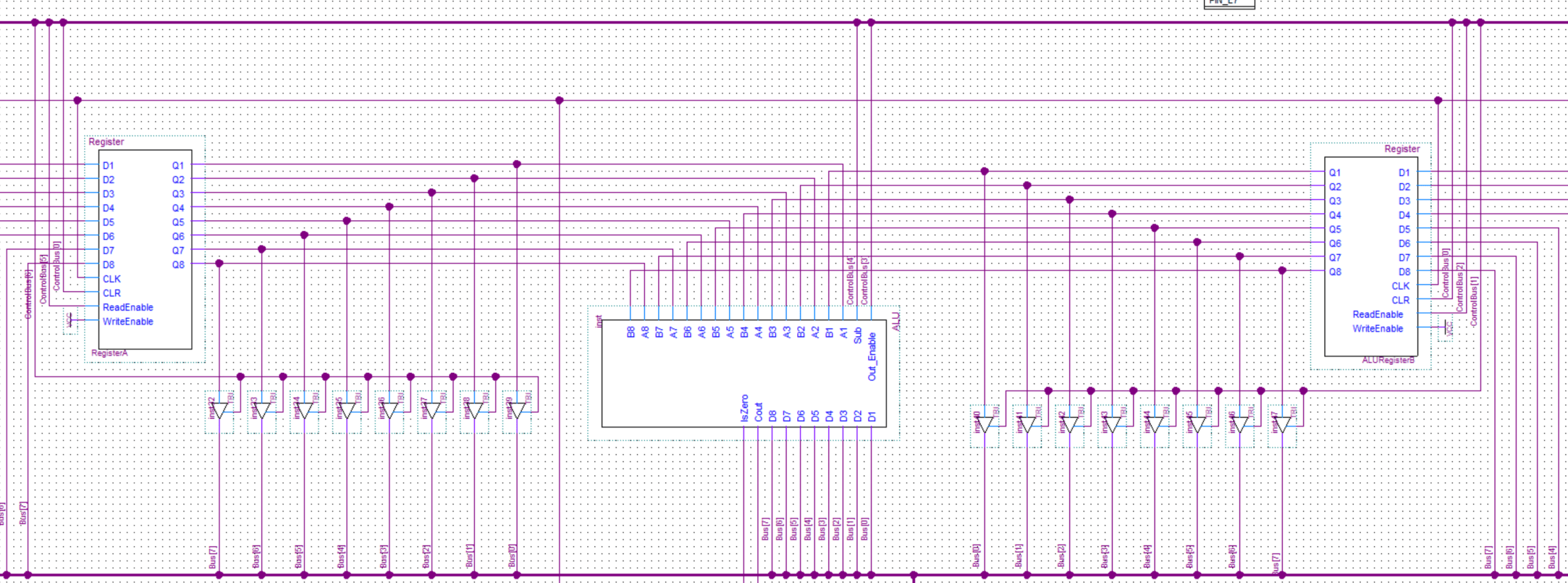# 8-Bit Computer ALU And The Flags RegisterShare:

In this post, I will talk about the ALU and the flags register of my 8-bit computer.

The arithmetical logical unit(ALU) is where all the computation happens in a computer. The ALU of this computer is very simple and only has the option to add or subtract numbers. So something like multiplication would have to be implemented in software.

The flags register stores the Carry(a carry occurs) and Is Zero(the result is a zero) flags that it receieves from the ALU.

## ALU

The two values(operands) will get stored in the A and B registers. After that, the values will be continuously output from the register into the ALU. The outputs of both registers are also connected to the BUS through some tristate buffers. The output of the ALU itself is connected straight to the BUS.

When bits from the A and B register arrive into the adder they are automatically added and the result is sent out of the Sum output. If the bits from both registers are 1 the C_Out output will be also set to 1. This output is thne feed into the C_In input of the next adder.

To implement the subtraction one of the values(B register) is XOR-ed and the carry in(C_In) of the first adder is set to 1. If you want to know how and why exactly this will perform subtraction(despite the fact that the bits are getting added) search more about “twos complement” or watch Ben Eaters video about it here.

Finally, there is a tristate buffer to control whether the value is put on the BUS or not.

This is the circuit for a half-adder. A half-adder can add two bits and output the sum and a carry bit. The reason it’s called a half adder is that it can’t take in the carry bit from the previous adder. We’ll see how that is implemented in the full-adder.
Supposing you already know how binary addition works: 00 -> 01 -> 10 -> 11 -> …  This is a textual description of the digital logic required to perform addition:
1. If A and B are 0 the Sum and Carry should both be 0.
2. If A or B is 1 the Sum is 1 and Carry is 0.
3. If both A and B are 1 the Sum is 0 and Carry is 1.
If you look at the truth table for the XOR gate you can see that it matches the logic required for the Sum output. And if you look at the truth table for the AND gate you can see that it matches the logic required for the Carry output.

A B C
0 0 0
0 1 1
1 0 1
1 1 0

#### AND Gate Truth Table

A B C
0 0 0
0 1 0
1 0 0
1 1 1
This should explain the circuit above. Now let’s look at the full-adder implementation.
A full-adder as the name implies is made from two half-adders chained together. The first half adder will add the bits from the two numbers(A and B). The second half-adder will add the sum output of the first half-adder and the carry bit from the previous full-adder(if present). It will then output the sum from the Sum output. The carry bits from both half-adders are connected to the carry out(C_Out) output through an OR gate.

### Flags Implementation

To get the Carry flag we simply connect the C_Out carry output of the Adder to the Cout output of the ALU. For the IsZero flag, we take the Sum outputs of every adder and put them into a NOR gate. The output of the gate is then connected to the IsZero output of the ALU.

## Flags Register

When the computation of the ALU is either zero the IsZero output of the ALU will output a 1 and when a carry occurs the Cout output of the ALU will output a 1. The result of these outputs will get stored into the flags register. The flags can then be used in the control unit for the JMZ(jump if zero) and JMC(jump if carry) instructions. See more about the implementation of that in the control unit in this post.
Share: